# How to Find the GCF | Methods and Examples

1. Math Lessons >
2. GCF

### What Is GCF?

GCF, the greatest common factor, is the largest number that evenly divides two or more numbers. It is also called HCF, the highest common factor or GCD, the greatest common divisor. There are various methods of finding the greatest common factor of a set of numbers. In this lesson, we will demonstrate three ways of finding the GCF.

### How Do You Find the GCF by Listing the Factors?

Listing the factors is a simple method used to find the GCF of smaller numbers. In this method, we list the factors of each number, pick out the common factors, and select the highest of those.

Let’s take the number pair 16 and 24.

What are the numbers that evenly divide 16?

We know 16 = 1 x 16, 16 = 2 x 8, and 16 = 4 x 4.

⇨ 16 has five factors: 1, 2, 4, 8, and 16.

Similarly, 24 = 1 x 24, 24 = 2 x 12, 24 = 3 x 8, and 24 = 4 x 6.

⇨ 24 has eight factors: 1, 2, 3, 4, 6, 8, 12, and 24.

Note: Make use of the divisibility rules to identify the factors of a number.

Now, we need to compare both lists and identify the common factors. You can choose to compare directly or use a Venn diagram for comparison. Here we have shown both.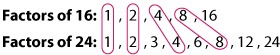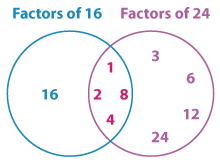Thus the common factors, or the factors depicted on the intersection, are 1, 2, 4, and 8.

Now, the greatest of the common factors is 8.

⇨ The GCF of 16 and 24 is 8.

Fast Fact 1

The greatest common factor of two prime numbers is 1.

For example, the GCF of (3, 7) is 1 as 3 and 7 have only one factor in common: 1.

Example 1

Determine the GCF of the number pair (12, 32).

Factors of 12: 1, 2, 3, 4, 6, 12

Factors of 32: 1, 2, 4, 8, 16, 32

⇨ GCF (12, 32) = 4.

Example 2

Find the GCF of 15 and 55 using Venn diagram.

The Venn diagram depicting the factors of 15 and 55 is: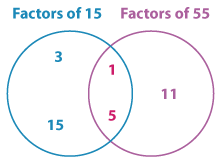The factors on the intersection are 1 and 5.

⇨ GCF (15, 55) = 5.

Example 3

What is the GCF of 13 and 29?

Here, 13 and 29 are prime numbers.

∴ GCF (13, 29) = 1.

### How Do You Find the GCF Using Prime Factorization?

Prime factorization is yet another technique for obtaining the greatest common factor of a number. This method is suitable for all numbers – smaller or larger. Unlike listing-the-factors method, prime factorization takes only the prime factors into account. All we need to do is:

Build prime factor trees for each number.

Express each number as a product of prime factors.

Multiply the factors in common to arrive at the GCF.

Let’s find the GCF of 18 and 27. The factor trees for the numbers are as shown: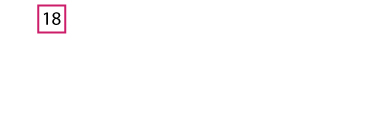Generate factor trees for 18 and 27 with a click on the number!

Now, expressing each number as a product of prime factors, we have: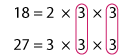As you can see, we have two 3s in common.

Thus, the GCF is the product of common factors: 3 x 3.

⇨ GCF (18, 27) = 9.

Example 1

Determine the GCF of 30 and 90 using prime factorization.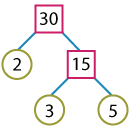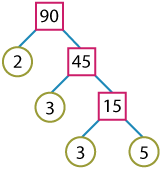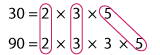The greatest common factor is 2 x 3 x 5.

⇨ GCF(30, 90) = 30.

Example 2

Find the GCF of 95 and 220 using prime factorization.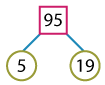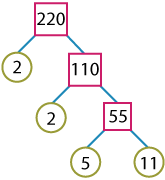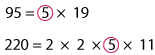⇨ GCF(95, 220) = 5.

Fast Fact 2

If in a number pair one number is the factor of the other, that number is the GCF.

In the above example, 30 is a factor of 90. So, the GCF of (30, 90) = 30.

### How Do You Find the GCF Using Repeated Division?

Repeated division is a quick and easy technique of finding the GCF of a set of numbers. This is also called successive division or the ladder method. This is an upside-down division that works by dividing both the numbers simultaneously by a common prime factor. The process stops when there are no prime factors in common.

Let’s find the GCF of 72 and 176 using division method.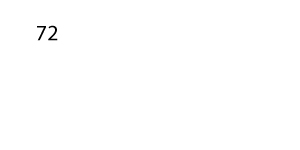Click on the number to view the repeated division of 72 and 176!

Now, the product of the common prime factors, 2 x 2 x 2 is the GCF.

⇨ GCF(72, 176) = 8.

Fast Fact 3

If the numbers do not have a common prime factor, their GCF is 1.

For example, the numbers 9 and 22 do not have any common prime factor.

⇨ GCF(9, 22) = 1.

Note: The number pairs that have 1 as their GCF are coprime.

Example 1

Calculate the GCF of 36 and 54 using successive division.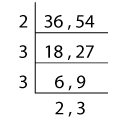Product of the common factors = 2 x 3 x 3

⇨ GCF(36, 54) = 18.

Example 2

Find the GCF of 448 and 504 using the ladder method.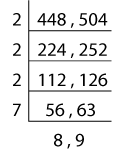Product of the common factors = 2 x 2 x 2 x 7

⇨ GCF(448, 504) = 56.

### GCF of More than Two Numbers

The GCF is not confined to a pair of numbers; we can find the GCF of multiple numbers also.

Let’s find the GCF of three numbers: 42, 56, and 98 using all three methods.

Listing the Factors

Factors of 42:
1, 2, 3, 6, 7, 14, 21, 42

Factors of 56:
1, 2, 4, 7, 8, 14, 28, 56

Factors of 98:
1, 2, 7, 14, 49, 98

GCF (42, 56, 98) = 14.

Prime Factorization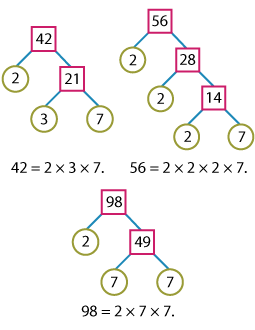Product of the common factors: 2 x 7

GCF (42, 56, 98) = 14.

Repeated Division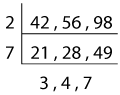Product of the common factors: 2 x 7

GCF (42, 56, 98) = 14.

Let’s press on! Here’s the set of four numbers that we’re going to find the GCF of.

126, 252, 162, 174

The numbers are a bit larger. So, let’s find their GCF using prime factorization and the ladder method.

Prime Factorization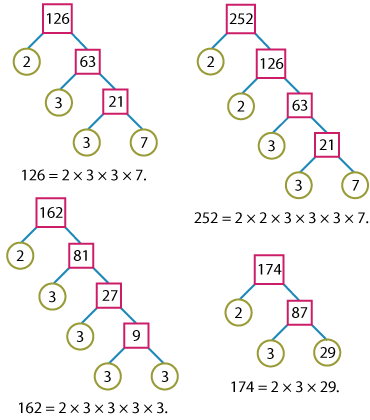Product of the common factors: 2 x 3

GCF (126, 252, 162, 174) = 6.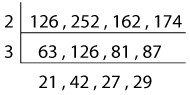Product of the common factors: 2 x 3

GCF (126, 252, 162, 174) = 6.

Thus no matter what method use, the GCF of a set of numbers is unique.

### GCF in Everyday Life

Let’s understand the application of GCF in everyday life with this scenario:

Irene is all set to celebrate her little artist’s birthday. She has 24 brushes and 36 assorted paint bottles to fill her goody bags with. Irene wants to distribute them evenly so each bag has the same number of brushes and paint bottles and no items are left over.

i) What is the greatest number of goody bags that Irene can make?

ii) How many paintbrushes and bottles will go into each goody bag?Irene has 24 paintbrushes and 36 paint bottles.

i) To find the number of goody bags that Irene can make with the same number of paintbrushes and paint bottles, we need to find the GCF of 24 and 36.

GCF Using Prime Factorization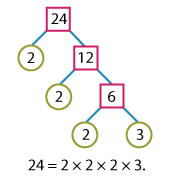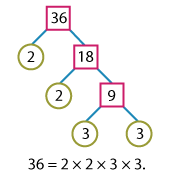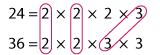The product of common factors: 2 x 2 x 3.

GCF(24, 36) = 12.

GCF Using Division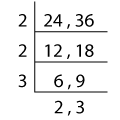The product of common factors: 2 x 2 x 3.

GCF(24, 36) = 12.

So the greatest number of goody bags that Irene can make is 12.

ii) Now to find out how many brushes and paint bottles go into each of the 12 goody bags, we need to divide 24 brushes evenly into 12 goody bags as shown below:

⇨ 24/12 = 2 paintbrushes each

And 36 paint bottles split into 12 goody bags will leave:

⇨ 36/12 = 3 paint bottles each

The goody bags are ready! That was pretty simple!

## Type the numbers in the text boxes, and calculate their GCF with a click of your mouse!

### The lesson in a Nutshell:

• The GCF of a set of numbers is the largest number that divides each number in the set.

• We can find the GCF of a set of numbers by listing the factors, by using prime factorization, or by performing repetitive division.

• To find the GCF by listing the factors,

• list the factors of each number,

• identify the common ones, and

• choose the greatest of those.

• To find the GCF using prime factorization,

• express each number as a product of prime factors,

• write down the common factors, and

• determine their product.

• To find the GCF using the ladder method,

• use the divisibility rules and divide the numbers simultaneously by a common factor,

• continue to divide until you end up with numbers that have no prime factors in common, and

• multiply the common factors.Pump up your practice with our free printable Greatest Common Factor (GCF) worksheets!

Progress
Score

Restart Quiz